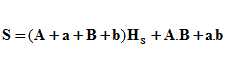Mathematics

Area/Volume of a Pyramidal Frustum

 Show figure Calculate:   Total Surface Area (S)  Lateral Surface Area (SL)  Volume (V)  Slant Height (Hs) h =Hs = A = B = a = b = To Memory 01 Memory 02 Memory 03 Memory 04 Memory 05 Memory 06 Replacing Adding Subtracting Multiplying Dividing
 Configurations Maximum of decimal places Unit of Measurement of Length: Impression Page Title (Optional) Comments to be printed (Optional)